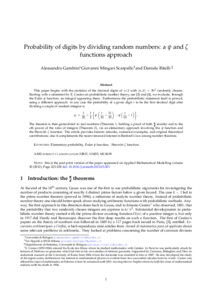# Probability of digits by dividing random numbers: a psi and zeta functions approach

Gambini, Alessandro ; Mingari Scarpello, Giovanni ; Ritelli, Daniele (2012) Probability of digits by dividing random numbers: a psi and zeta functions approach. Expositiones Mathematicae, 30 (3). p. 223238. ISSN 0723-0869
Full text available as:Preview Download (1MB) | Preview

## Abstract

This paper begins with the statistics of the decimal digits of n/d with n, d randomly chosen. Starting with a statement by E. Cesàro on probabilistic number theory we evaluate, through the Euler psi function, an integral appearing there. Furthermore the probabilistic statement itself is proved, using a different approach.The theorem is then generalized to real numbers (Theorem 1) and to the alpha-th power of the ratio of integers (Theorem 2), via an elementary approach involving the psi function and the Hurwitz zeta function. The article provides historic remarks, numerical examples, and original theoretical contributions: also it complements the recent renewed interest in Benford's law among number theorists.

Abstract
Document type
Article
Creators
CreatorsAffiliationORCID
Gambini, Alessandro
Mingari Scarpello, Giovanni
Ritelli, Daniele
Keywords
Elementary probability, Euler psi function, Hurwitz zeta function
Subjects
ISSN
0723-0869
DOI
Deposit date
31 Oct 2012 09:57